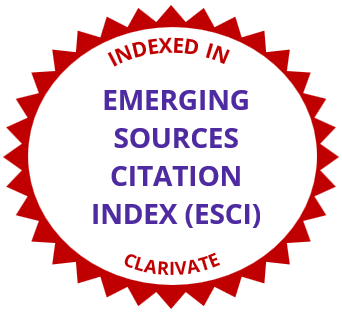# International Electronic Journal of Mathematics EducationDidactic Effectiveness of Mathematical Definitions: The Case of the Absolute Value
• Article Type: Research Article
• International Electronic Journal of Mathematics Education, 2007 - Volume 2 Issue 2, pp. 72-90
https://doi.org/10.29333/iejme/176
• Published Online: 06 Jul 2007

# Abstract

Quite often a mathematical object may be introduced by a set of equivalent definitions. A fundamental question is determining the “didactic effectiveness” of the techniques for solving a kind of problem associated with these definitions; this effectiveness is evaluated by taking into account the epistemic, cognitive and instructional dimensions of the study processes. So as to provide an example of this process, in this article we study the didactic effectiveness of techniques associated with different definitions of absolute value notion. The teaching and learning of absolute value are problematic; this is proven by the amount and heterogeneity of research papers that have been published. We propose a “global” study from an ontological and semiotic point of view.

# References

• Arcidiacono, M. J. (1983). A visual approach to absolute value. The Mathematics Teacher, 76 (3), 197?201.
• Barrow, R. (1997). Language: definition and metaphor. Studies in Philosophy and Education, 16, 113?124.
• Bills, L., and Tall, D.(1998). Operable definitions in advanced mathematics: the case of the least upper bound. In A. Olivier and K. Newstead (eds.), Proceedings of PME 22 (Stellenbosch, South Africa), Vol. 2, 104?111.
• Brown, J. R. (1998). What is a definition?. Foundations of Science, 1, 111?132.
• Chiarugi, I., Fracassina, G., and Furinghetti, F. (1990). Learning difficulties behind the notion of absolute value. Proceedings of PME 14 (México), Vol. 3, 231?238.
• De Villiers, M.(1998). To teach definitions in geometry or teach to define?. In A. Olivier and K. Newstead (eds.), Proceedings of PME 22 (Stellenbosch, South Africa), Vol. 2, 248?255.
• Gagatsis, A. (2003). A multidimensional approach to understanding and learning mathematics. In Gagatsis and Papastavridis (eds), Proceedings of the 3rd International Mediterranean Conference on Mathematics Education. (pp. 53–72). Athènes: Hellas.
• Gagatsis, A., and Thomaidis, I. (1994). Un étude multidimensionnelle du concept de valeur absolue. In M. Artigue, R. Gras, C. Laborde and P. Tavignot (eds.), Vingt ans de didactique de mathematiques en France. (pp. 343?348). Grenoble: La Pensée Sauvage.
• Godino, J. D. (2002). Un enfoque ontológico y semiótico de la cognición matemática. Recherche en Didactique des Mathématiques, 22(2/3), 237?284.
• Godino, J. D. and Batanero, C. (1998). Clarifying the meaning of mathematical objects as a priority area of research in mathematics education. In A. Sierpinska and J. Kilpatrick (Eds.), Mathematics Education as a Research Domain: A Search for Identity (pp. 177-195). Dordrecht: Kluwer, A. P.
• Godino, J. D., Batanero, C., and Roa, R. (2005). An onto-semiotic analysis of combinatorial problems and the solving processes by university students. Educational Studies in Mathematics, 60 (1), 3 - 36.
• Gras, R. (1996). L’implication statistique. Grenoble, FRA: La Pensée Sauvage.
• Leithold, L. (1968). The calculus with analytic geometry. New York: Harper & Row.
• Horak, V. M. (1994). Investigating absolute-value equations with the graphing calculator. The Mathematics Teacher, 87(1), 9?11.
• Leikin, R., and Winicki-Landman, G. (2000). On equivalent and non-equivalent definitions: part 2. For the Learning of Mathematics, 20(2), 24?29.
• Linchevsky, L., Vinner, S., and Karsenty, R. (1992). To be or not to be minimal? Student teachers’ views about definitions in geometry. Proceedings of PME 16 (Durham, NH), Vol. 2, 48?55.
• Mariotti, M. A., and Fischbein, E. (1997). Defining in classroom activities. Educational Studies in Mathematics, 34, 219?248.
• Mollin, R. A. (1998). Fundamental number theory with applications. Boca Raton, Florida: CRC Press.
• Perrin-Glorian, M-J. (1995). The absolute value in secondary school. A case study of “Institutionalisation” process. Proceedings of PME 19 (Recife, Brazil), Vol. 2, 74?81.
• Tall, D. (1991). The psychology of advanced mathematical thinking. In D. Tall (ed.), Advanced Mathematical Thinking (pp. 3?21). Dordrecht, HOL: Kluwer.
• Ullman, S. (1962). Semántica. Introducción a la ciencia del significado. Aguilar, Madrid, 1978.
• Vinner, S. (1991). The role of definitions in the teaching and learning of mathematics. In D. Tall (ed.), Advanced Mathematical Thinking (pp. 65?81). Dordrecht: Kluwer.
• Williams, R. L. (1999). Operational definitions and assessment of higher-order cognitive constructs. Educational Psychology Review, 11(4), 411?427.
• Winicki-Landman, G., and Leikin, R. (2000). On equivalent and non-equivalent definitions: part 1. For the Learning of Mathematics, 20(1), 17?21.